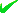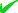News By Tag
Industry News
News By Location

Country(s)

Industry News

December 2019
FrThWeTuMoSuSa
13121110987

# The Different Types of Interest And Terminology Winston Rowe And Associates

It can be confusing at times with all of the financial jargon associated with taking out a loan, particularly when all you really want to know is exactly how much it is going to cost you on a monthly basis.
By: Winston Rowe and Associates

Listed Under

Industry:
* Banking

Location:
* Los Angeles - California - US

LOS ANGELES - Nov. 18, 2018 - PRLog -- There is much to be gained from having a basic understanding about interest rates, the different types of interest rates that are available, and how interest rates are calculated before you enter into any loan arrangement.

The more you know about interest rate formulas the better you'll be positioned to make a more informed judgement when it comes to taking out a loan and, in doing so, ensure that you keep as much of your money in your pocket as possible.

What is 'interest'?

In its simplest form, 'interest' is the cost of borrowing money, and it is normally expressed in terms of a percentage of the overall loan. Not only will you have to pay back the original amount of money borrowed (the principal), but you'll also have to pay back the cost of borrowing that money (the interest, plus any setting up fees etc.)

How much interest you have to pay on any given loan is subject to a number of different factors, depending on which lending institution you borrow the money from and the terms of the loan.

Fixed Rate Interest

Fixed rate interest is simply as the name suggests: a 'fixed' percentage of the loan must be paid back during the life of the loan. For example (using dollars as our currency), a \$1,000 loan with a fixed rate of interest of 5% per annum, means that if the loan amount were to be paid off in 12 months, the total amount the borrower would pay back would be \$1050.

Fixed rate interest loans make it very easy to calculate the exact amount of money the borrower will have to pay back each month as the amount never changes.

Variable Rate Interest

Variable rate interest loans allow the lender to set the interest rate to whatever market conditions demand at any given time during the life of the loan.

The attraction of variable interest rate loans is that you can benefit from any future drop in market interest rates when your monthly repayments are reduced to reflect the lower interest rate.

However, the opposite also holds true. If the market decides it's time for interest rates to rise, so too will your repayments. Mortgage loans, for example, are mostly set up with a variable interest rate, as it is virtually impossible to predict market conditions years ahead. In many cases you can opt for a fixed rate for a few years but after this period the loan reverts to a variable rate (these deals vary from lender to lender).

Make sure you fully understand the consequences of a variable interest rate loan if you are considering taking one out. If interest rates rise dramatically you could find yourself in financial difficulties.

APR

APR, or 'Annual Percentage Rate' is the total cost of the loan based on a yearly metric. In most cases this includes set up fees, administration costs and so on. In many countries, financial lenders must disclose the APR so that consumers have the chance to measure all lenders against a common metric.

Simple Interest

Interest rates are seldom calculated using the simple interest rate formula but rather are more likely to be calculated using the compound interest formula.

However, to explain, simple interest is calculated by multiplying the loan amount (e.g. \$1000) by the interest rate (e.g. 5%) by the number of payment periods over the life of the loan (e.g. 24 months).

The thing to keep in mind here is that the interest rate may be expressed in terms of an annual rate, e.g. 5% per annum, whereas the payment periods might be expressed in months, e.g. 24 months. To ensure your calculation of simple interest is accurate, you need to make sure that both the interest rate and the payment periods are expressed in the same manner, say annually or monthly.

For example, using the figures above our \$1000 loan would be at 5% per annum, and taken out over just 2 years (as opposed to 24 months). So here the calculation would be 1000 x 0.05 x 2 (loan x interest x term) = 100. So, the amount of simple interest that we would pay on this loan over the Two year term would be \$100.

Compound Interest

Compound interest relates to charges the borrower must pay not just on the principal amount borrowed, as in simple interest, but also on any interest outstanding at that point in time.

To illustrate the difference between compounding interest and simple interest, consider the following (very simplified) scenario of a \$1000 loan taken out at 10% over 2 years (assuming no monthly payments are made on the loan):

Example of Simple vs Compound Interest

Simple Interest:

First year: \$1,000 x 1 year x 10% = \$100 in interest

Second year: \$1,000 x 1 year x 10% = \$100 in interest

Total Interest: \$200

Total of the principal amount plus interest = \$1,200

In this scenario, the total amount of interest paid over the life of the loan would be \$200

Compound Interest:

First year: \$1,000 x 1 year x 10% = \$100 in interest

Second year: \$1,100 (\$1000 principal plus \$100 accrued interest) x 1 year x 10% = \$110 in interest

Total Interest: \$210

Total of the principal amount plus interest = \$1,210

In this scenario, with interest compounded annually, the total amount of interest paid is \$210

his is a very simplified example but it should be enough to highlight the workings of compound interest.

Published by Winston Rowe and Associates, a national consulting firm for commercial real estate. They can be contacted at 248-246-2243 or visit them online at http://www.winstonrowe.com

Contact
Staff Writer
***@winstonrowe.com
End
Email Tags : ***@winstonrowe.com: How To Calculate Interest : Banking : Los Angeles - California - United States
Account Email AddressAccount Phone NumberDisclaimer     Report Abuse